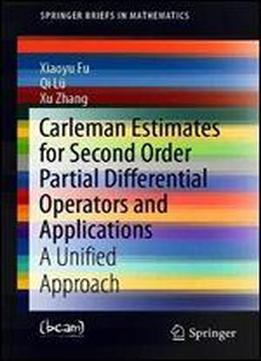# Carleman Estimates For Second Order Partial Differential Operators And Applications: A Unified Approach by Xiaoyu Fu / 2019 / English / PDF, EPUB

This book provides a brief, self-contained introduction to Carleman estimates for three typical second order partial differential equations, namely elliptic, parabolic, and hyperbolic equations, and their typical applications in control, unique continuation, and inverse problems. There are three particularly important and novel features of the book. First, only some basic calculus is needed in order to obtain the main results presented, though some elementary knowledge of functional analysis and partial differential equations will be helpful in understanding them. Second, all Carleman estimates in the book are derived from a fundamental identity for a second order partial differential operator the only difference is the choice of weight functions. Third, only rather weak smoothness and/or integrability conditions are needed for the coefficients appearing in the equations. Carleman Estimates for Second Order Partial Differential Operators and Applications will be of interest to all researchers in the field.

views: 270